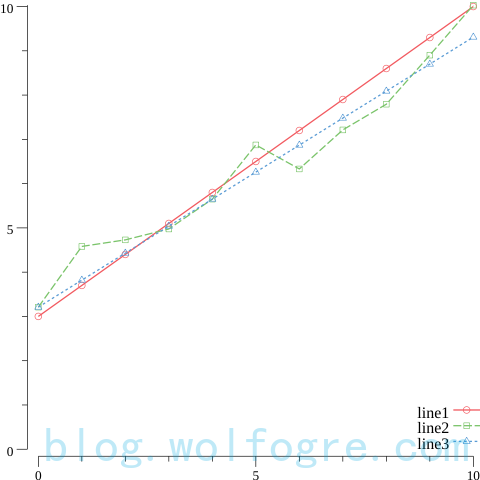# 从二维绘图到数值优化——初探 golang 科学计算包 gonum

## gonum 简介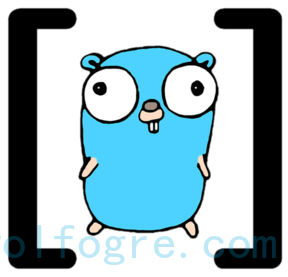gonum 是一个用 golang 写的数学工具包，包括矩阵计算、数理统计、数值优化等等。GitHub 上，目前该项目拥有 3.4k 的 stars，超过 5000 次提交，并且仍保持着活跃更新。

gonum 项目所属的组织也叫 Gonum（此处故意大写，方便区分），这个组织下还有一个独立的包 plot，用于数据可视化，而脱离可视化去使用 gonum 是很枯燥的，所以若无特别说名，本文说的“gonum 包”，实际上是指 Gonum 组织下 gonum、plot 等包的集合，而不是单指 gonum 这一个包。

Gonum 有一个官网：www.gonum.org，但很遗憾目前网站上并没有多少实质性的内容，也没相关的教程或文档，从网上找到的一些资料不仅不多，而且老旧的很，与新版的代码不相符。

## 画些点线

``````go get -u gonum.org/v1/gonum/...
``````

``````package main

import (
"gonum.org/v1/plot"
"gonum.org/v1/plot/plotter"
"gonum.org/v1/plot/plotutil"
"gonum.org/v1/plot/vg"
)

func main() {
var a, b float64 = 0.7, 3
points := plotter.XYs{}
for i := 0; i <= 10; i++ {
points = append(points, plotter.XY{
X: float64(i),
Y: a*float64(i) + b,
})
}

plt, err := plot.New()
if err != nil {
panic(err)
}
plt.Y.Min, plt.X.Min, plt.Y.Max, plt.X.Max = 0, 0, 10, 10

"line1", points,
); err != nil {
panic(err)
}

if err := plt.Save(5*vg.Inch, 5*vg.Inch, "01-draw-line.png"); err != nil {
panic(err)
}
}
// 完整代码： https://github.com/wolfogre/gonum-example/blob/master/01-draw-line/main.go
``````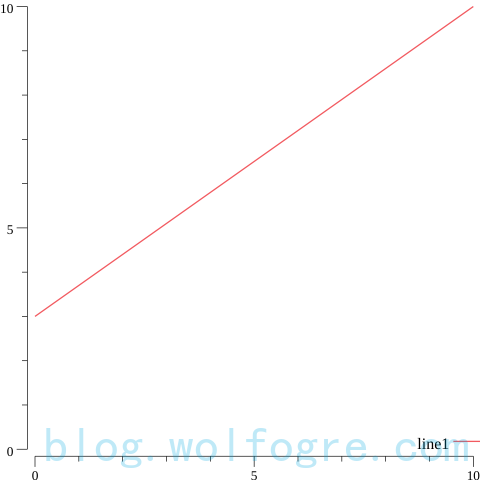1. 类型 `plotter.XYs` 可以方便描述一组二维向量，即一个 `plotter.XY` 数组，顾名思义，`plotter.XY` 拥有 X 和 Y 两个字段；
2. `plot.New()` 可以获得一个二维坐标系，我们可以通过一些可选操作来设置坐标系各个轴的最大值和最小值，帮助图像展示得更合理；
3. plotutil 是个辅助工具，可以帮助我们将 `plotter.XYs` 转换为 Line 并绘制到坐标系上，当然我们也可以不借助它，自己构造 `plotter.Line` 对象并 `Add` 到坐标系上；
4. 由于 golang 尚没有成熟的 GUI 组件，或者说 gonum 目前没有集成任何 GUI 组件，所以如果我们想看到绘图结果，需要将 `plot.Plot` 对象保存成图像文件查看。

``````// ...

func main() {
var a, b float64 = 0.7, 3
points1 := plotter.XYs{}
points2 := plotter.XYs{}

for i := 0; i <= 10; i++ {
points1 = append(points1, plotter.XY{
X: float64(i),
Y: a*float64(i) + b,
})
points2 = append(points2, plotter.XY{
X: float64(i),
Y: a*float64(i) + b + (2*rand.Float64() - 1),
})
}

// ...

"line1", points1,
"line2", points2,
); err != nil {
panic(err)
}

// ...
}
// 完整代码：https://github.com/wolfogre/gonum-example/blob/master/02-draw-linepoint/main.go
``````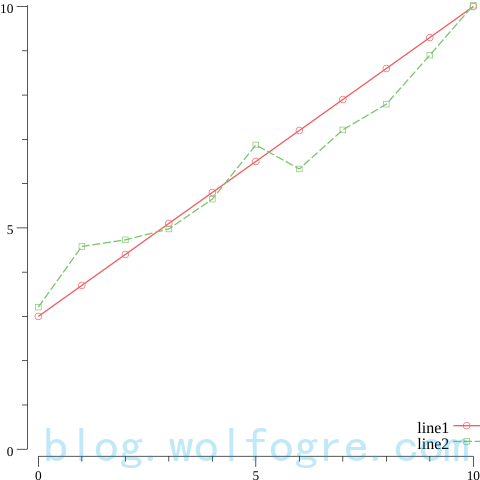``````// ...

func main() {
// ...

fa, fb := LeastSquares(points2)
points3 := plotter.XYs{}
for i := 0; i <= 10; i++ {
points3 = append(points3, plotter.XY{
X: float64(i),
Y: fa*float64(i) + fb,
})
}

// ...

"line1", points1,
"line2", points2,
"line3", points3,
); err != nil {
panic(err)
}

// ...
}

func LeastSquares(points plotter.XYs) (a, b float64) {
var xSum, ySum float64
for _, point := range points {
xSum += point.X
ySum += point.Y
}
xAvg, yAvg := xSum/float64(points.Len()), ySum/float64(points.Len())

var xySum, xxSum float64
for _, point := range points {
xySum += (point.X - xAvg) * (point.Y - yAvg)
xxSum += (point.X - xAvg) * (point.X - xAvg)
}

a = xySum / xxSum
b = yAvg - a*xAvg
return
}
// 完整代码：https://github.com/wolfogre/gonum-example/blob/master/03-least-squares/main.go
``````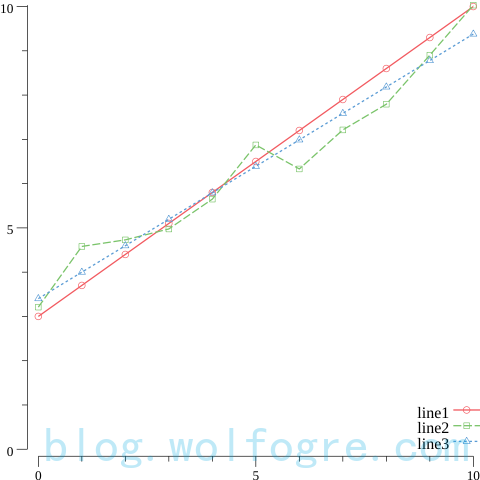## 画热图

``````// ...

func main() {
points := plotter.XYs{}
for i := 0; i < 10; i++ {
points = append(points, plotter.XY{
X: 100 * rand.Float64(),
Y: 100 * rand.Float64(),
})
}

scatter, err := plotter.NewScatter(points)
if err != nil {
panic(err)
}
scatter.Shape = draw.CircleGlyph{}

plt, err := plot.New()
if err != nil {
panic(err)
}
plt.Y.Min, plt.X.Min, plt.Y.Max, plt.X.Max = 0, 0, 100, 100

// ...
}
// 完整代码：https://github.com/wolfogre/gonum-example/blob/master/04-draw-dot/main.go
``````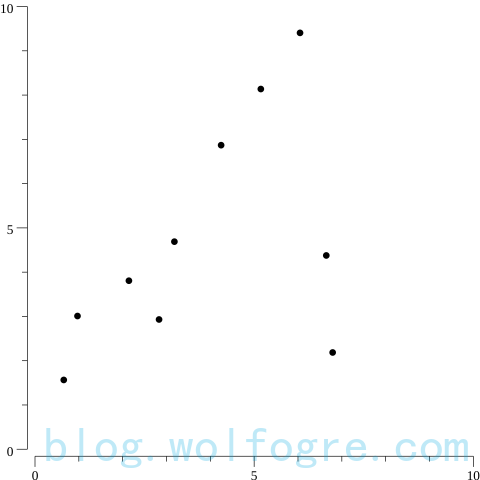``````// ...

func main() {
// ...

heatmap := plotter.NewHeatMap(Heat(points), moreland.SmoothBlueRed().Palette(100))

// ...

// ...
}

type Heat plotter.XYs

func (h Heat) Dims() (c, r int) { return 100, 100 }
func (h Heat) X(c int) float64  { return float64(c) }
func (h Heat) Y(r int) float64  { return float64(r) }
func (h Heat) Z(c, r int) float64 {
var sum float64
for _, p := range h {
sum += math.Sqrt(math.Pow(p.X-h.X(c), 2) + math.Pow(p.Y-h.Y(r), 2))
}
return -sum
}
``````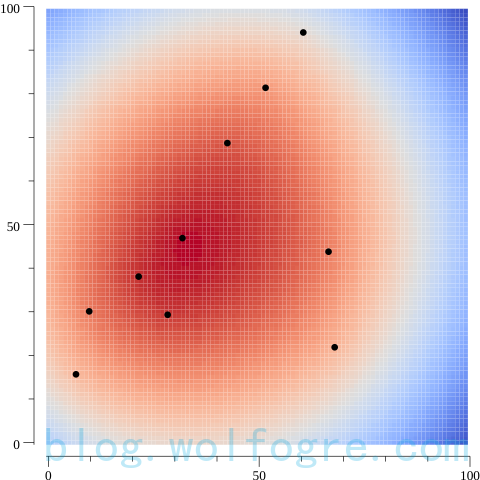## 描述问题

``````type Problem struct {
Func func(x []float64) float64
Hess func(hess *mat.SymDense, x []float64)
Status func() (Status, error)
}
``````

`Grad` 存放的是代价函数对应的导数，严格来说，这里叫“梯度”才更准确，这里不细究，可以用导数来理解。可想而知，梯度的方向指示了极值的所在的相对方向，有了函数对应的梯度，在求解的过程中可以更快地收敛到极值。

`Hess` 存放的是代价函数的黑塞矩阵，关于黑塞矩阵是什么可以看维基百科的解释，坦白说，我看了半天也没看懂，但简单理解就是，它也是为了帮助某些数值优化算法，一般指牛顿法，更好更快地找到极值。

``````// ...

func main() {
// ...

Func := func(x []float64) float64 {
if len(x) != 2 {
panic("illegal x")
}
var sum float64
for _, point := range points {
sum += math.Sqrt(math.Pow(point.X-x, 2) + math.Pow(point.Y-x, 2))
}
return sum
}

problem := optimize.Problem{
Func:   Func,
Hess:   nil,
Status: nil,
}

_ = problem
}
// 完整代码：https://github.com/wolfogre/gonum-example/blob/master/06-problem/main.go
``````

## 下山单纯形法

``````// ...

func main() {
// ...

Func := func(x []float64) float64 {
if len(x) != 2 {
panic("illegal x")
}
var sum float64
for _, point := range points {
sum += math.Sqrt(math.Pow(point.X-x, 2) + math.Pow(point.Y-x, 2))
}
return sum
}

problem := optimize.Problem{
Func: Func,
}

result, err := optimize.Minimize(problem, []float64{1, 1}, &optimize.Settings{}, &optimize.NelderMead{})
if err != nil {
panic(err)
}

aim, err := plotter.NewScatter(plotter.XYs{{
X: result.X,
Y: result.X,
}})
if err != nil {
panic(err)
}
aim.Shape = draw.CircleGlyph{}
aim.Color = color.White

// ...

// ...
}

// ...
``````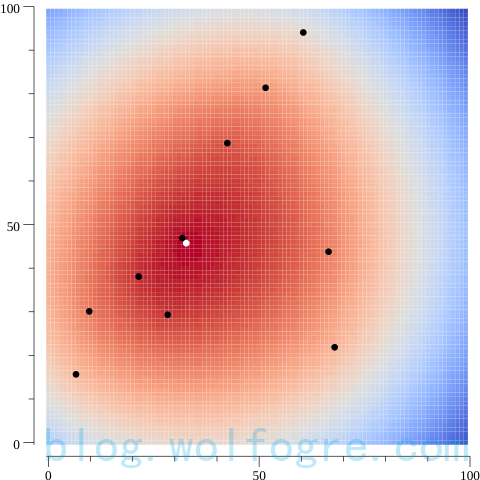``````func optimize.Minimize(p Problem, initX []float64, settings *Settings, method Method) (*Result, error)
``````

• `p Problem`：求解的数值优化问题，这里就是“蚂蚁寻热”的问题；
• `initX []float64`：初始坐标，即从什么位置开始搜索最优解，这里是随便给的 (1, 1)；
• `settings *Settings`：一些求解过程中的可选配置，这里给的是空配置，即用默认配置；
• `method Method`：用什么数值优化算法来求解问题，这里给的是 `optimize.NelderMead`，即 gonum 自带的下山单纯形法实现。

## 图示求解过程

``````// ...

func main() {
// ...

recorder := &Recorder{}

result, err := optimize.Minimize(problem, []float64{1, 1}, &optimize.Settings{
Recorder: recorder,
if err != nil {
panic(err)
}

pathLines, pathPoints, err := plotter.NewLinePoints(recorder.XYs)
if err != nil {
panic(err)
}

// ...

// ...
}

// ...

type Recorder struct {
XYs plotter.XYs
}

func (r *Recorder) Init() error {
return nil
}

func (r *Recorder) Record(location *optimize.Location, op optimize.Operation, _ *optimize.Stats) error {
if op != optimize.MajorIteration && op != optimize.InitIteration && op != optimize.PostIteration {
return nil
}
r.XYs = append(r.XYs, plotter.XY{
X: location.X,
Y: location.X,
})
return nil
}
``````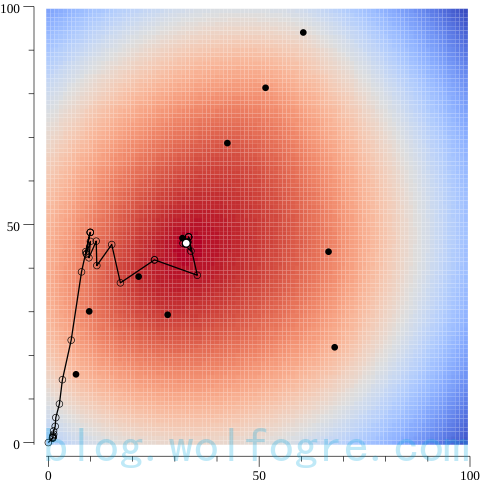## 使用其他算法

`BFGS` 需要 需要 不需要
`CG` 需要 需要 不需要
`CmaEsChol` 需要 不需要 不需要
`GradientDescent` 需要 需要 不需要
`GuessAndCheck` 需要 不需要 不需要
`LBFGS` 需要 需要 不需要
`ListSearch` 需要 不需要 不需要
`NelderMead` 需要 不需要 不需要
`Newton` 需要 需要 需要

``````// ...

func main() {
// ...

Func := func(x []float64) float64 {
if len(x) != 2 {
panic("illegal x")
}
var sum float64
for _, point := range points {
sum += math.Sqrt(math.Pow(point.X-x, 2) + math.Pow(point.Y-x, 2))
}
return sum
}
}
delta := 1e-9
for i, v := range x {
x[i] = v - delta
f1 := Func(x)
x[i] = v + delta
f2 := Func(x)
x[i] = v
grad[i] = (f2 - f1) / (2 * delta)
}
}

problem := optimize.Problem{
Func: Func,
}

methods := []struct {
Name   string
Method optimize.Method
}{
{"BFGS", &optimize.BFGS{}},
{"CG", &optimize.CG{}},
{"CmaEsChol", &optimize.CmaEsChol{}},
{"GuessAndCheck", &optimize.GuessAndCheck{
Rander: distmv.NewUniform([]r1.Interval{{0, 100}, {0, 100}}, xrand.NewSource(0)),
}},
{"LBFGS", &optimize.LBFGS{}},
{"ListSearch", &optimize.ListSearch{
Locs: mat.NewDense(6, 2, []float64{
0, 10,
20, 30,
40, 50,
60, 70,
80, 90,
90, 100,
}),
}},
//{"Newton", &optimize.Newton{}}, // what a pity
}

for _, method := range methods {
recorder := &Recorder{}

result, err := optimize.Minimize(problem, []float64{1, 1}, &optimize.Settings{
Recorder: recorder,
}, method.Method)
if err != nil {
panic(err)
}

// ...
}
}

// ...
// 完整代码：https://github.com/wolfogre/gonum-example/blob/master/09-other-methods/main.go
``````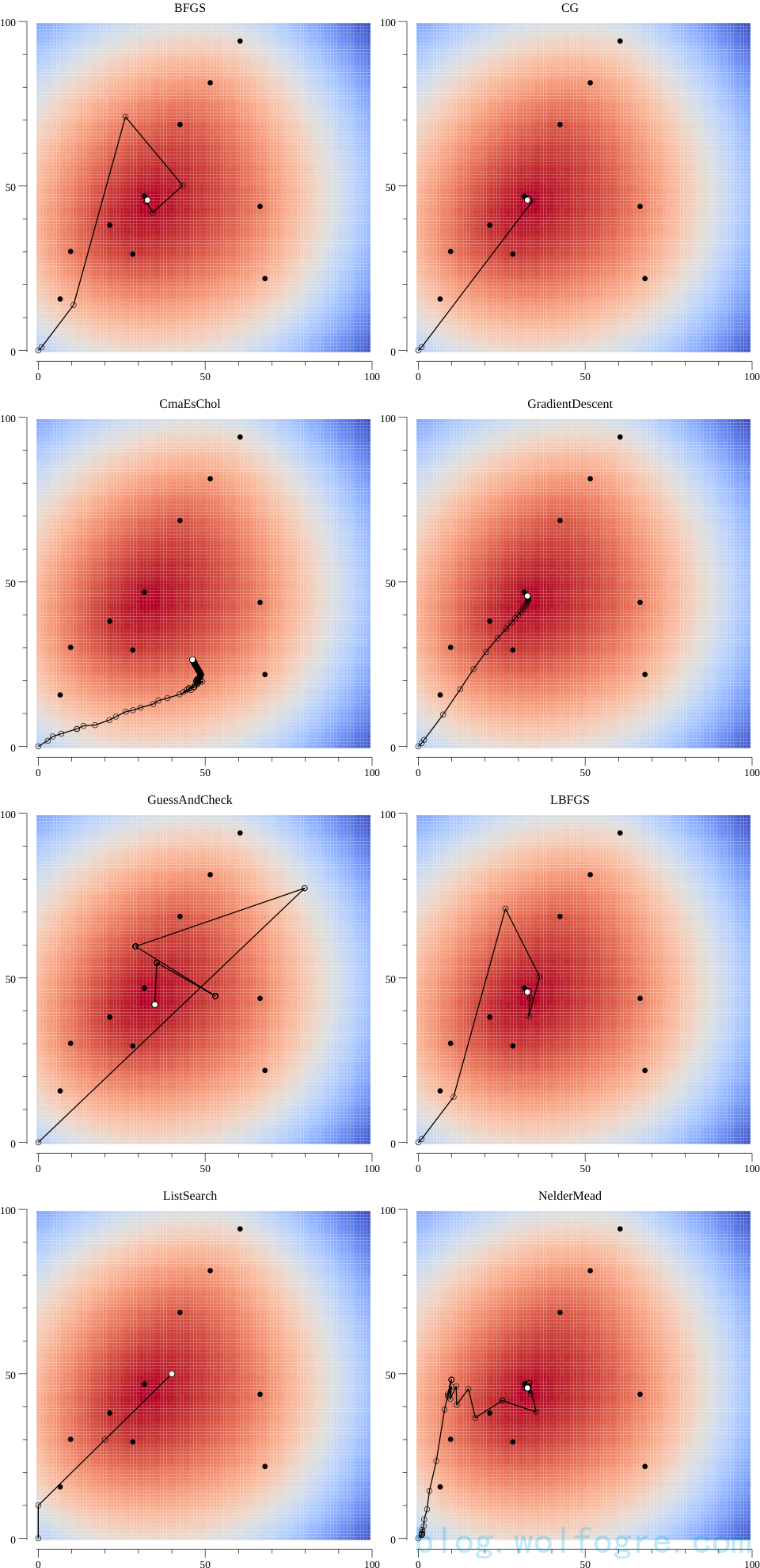## 用数值优化来回归

``````// ...

func main() {
var a, b float64 = 0.7, 3
points1 := plotter.XYs{}
points2 := plotter.XYs{}

for i := 0; i <= 10; i++ {
points1 = append(points1, plotter.XY{
X: float64(i),
Y: a*float64(i) + b,
})
points2 = append(points2, plotter.XY{
X: float64(i),
Y: a*float64(i) + b + (2*rand.Float64() - 1),
})
}

result, err := optimize.Minimize(optimize.Problem{
Func: func(x []float64) float64 {
if len(x) != 2 {
panic("illegal x")
}
a := x
b := x
var sum float64
for _, point := range points2 {
y := a*point.X + b
sum += math.Abs(y - point.Y)
}
return sum
},
if err != nil {
panic(err)
}

fa, fb := result.X, result.X
points3 := plotter.XYs{}
for i := 0; i <= 10; i++ {
points3 = append(points3, plotter.XY{
X: float64(i),
Y: fa*float64(i) + fb,
})
}

// ...
}
// 完整代码：https://github.com/wolfogre/gonum-example/blob/master/10-optimize-fit/main.go
``````